Ch.15 - Acid and Base EquilibriumWorksheetSee all chapters
 Ch.1 - Intro to General Chemistry 2hrs & 53mins 0% complete Worksheet Ch.2 - Atoms & Elements 2hrs & 49mins 0% complete Worksheet Ch.3 - Chemical Reactions 3hrs & 25mins 0% complete Worksheet BONUS: Lab Techniques and Procedures 1hr & 38mins 0% complete Worksheet BONUS: Mathematical Operations and Functions 47mins 0% complete Worksheet Ch.4 - Chemical Quantities & Aqueous Reactions 3hrs & 30mins 0% complete Worksheet Ch.5 - Gases 3hrs & 47mins 0% complete Worksheet Ch.6 - Thermochemistry 2hrs & 28mins 0% complete Worksheet Ch.7 - Quantum Mechanics 2hrs & 35mins 0% complete Worksheet Ch.8 - Periodic Properties of the Elements 1hr & 57mins 0% complete Worksheet Ch.9 - Bonding & Molecular Structure 2hrs & 5mins 0% complete Worksheet Ch.10 - Molecular Shapes & Valence Bond Theory 1hr & 31mins 0% complete Worksheet Ch.11 - Liquids, Solids & Intermolecular Forces 3hrs & 40mins 0% complete Worksheet Ch.12 - Solutions 2hrs & 17mins 0% complete Worksheet Ch.13 - Chemical Kinetics 2hrs & 22mins 0% complete Worksheet Ch.14 - Chemical Equilibrium 2hrs & 26mins 0% complete Worksheet Ch.15 - Acid and Base Equilibrium 4hrs & 42mins 0% complete Worksheet Ch.16 - Aqueous Equilibrium 3hrs & 48mins 0% complete Worksheet Ch. 17 - Chemical Thermodynamics 1hr & 44mins 0% complete Worksheet Ch.18 - Electrochemistry 2hrs & 58mins 0% complete Worksheet Ch.19 - Nuclear Chemistry 1hr & 33mins 0% complete Worksheet Ch.20 - Organic Chemistry 3hrs 0% complete Worksheet Ch.22 - Chemistry of the Nonmetals 2hrs & 1min 0% complete Worksheet Ch.23 - Transition Metals and Coordination Compounds 1hr & 54mins 0% complete Worksheet

# pH and pOH

See all sections
###### pH vs. pOH

The pH and pOH of a compound helps to determine its acidity and basicity.

###### The pH Scale

Concept #1: Understanding pH vs. pOH.

Transcript

Hey guys, in this new video we’re going to take a closer look at pH and pOH. We’re going to say that when it comes to the concentrations of H+ and OH-, they usually come as very small numbers. What we need to realize here is that the pH scale was invented to basically turn these varying small numbers into more manageable ones. We’re going to say under normal conditions, the pH scale operates within the range of 0 to 14. But what we need to realize here is that the pH scale could be less than 0 or could be greater than 14. All we would need to do is increase the concentration of our acid or base. Remember, 0 to 14 are not the only numbers that your pH or pOH could be. They could be greater or less than that. It all again depends on upping the concentration of your compound. If you make your concentration very high like over 1 molar, then it will give you a very high or very low pH.
We’re going to say that by taking the negative log of H+ and OH-, we can find pH and pOH. pH just means negative log of H+ concentration or negative log of H3O+. Because remember we said this before H+, H3O+ same exact thing. pOH is the negative log of OH-, so that means that p represents negative log.

pH stands for the negative logarithmic function of your hydronium concentration, whereas pOH stands for the negative logarithmic function of hydroxide concentration.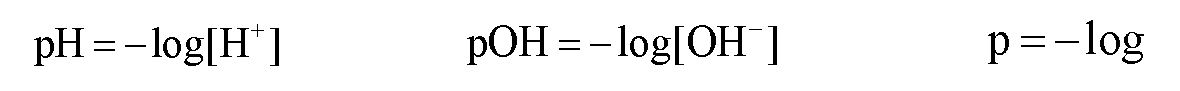Concept #2: The connection to Hydronium ion and Hydroxide ion concentrations.

Transcript

What we should realize here is that by recognizing the relationship between H+ and OH-, we can find a new way to express them in terms of pH and pOH. We come back here. We say that pH equals negative log of H+. We could divide both sides by -1 to get rid of this negative, so –pH equals a log of H+. We want to just isolate the H+ so we’re going to divide out the log. When you divide anything by log, it becomes 10 to that whatever the variable is.
We just found a new relationship. We can say that H+, the concentration of H+ ions is equal to 10 to the negative pH. We could do the same thing with pOH. Divide both sides by -1 where -pOH equals log of OH-. Divide both sides by log, so OH- equals 10 to the negative pOH. So, just remember the relationship that we have here with these first two equations and how we have the relationship with their concentrations. Because sometimes you’ll be asked to find H+ concentration if they give you pH. Sometimes you may be asked to find OH- concentration if they give you pOH. You have to know the relationship both frontwards and backwards.

If we know the pH or pOH concentration then we can determine the concentration of hydronium ions or hydroxide ions.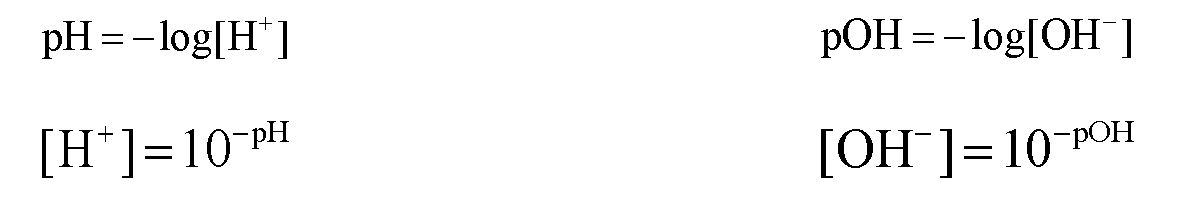Concept #3: The pH Scale.

Transcript

We’re going to say a species with a pH greater than 7 is classified as basic. If you’re basic, you’re going to say that your H+ concentration is less than your OH- concentration. We can also say that the stronger the base, then the higher the pH. The higher the pH, the greater your hydroxide concentration. We can understand this relationship as strongest base would have the highest pH and therefore have the highest OH- concentration. But remember, we already went over this several videos ago. How do we determine if something is weak or strong? You still need to know those in order to do the following questions because again, I’ve said it so many times before, why do we need to know the strength of things? Because eventually we’re going to have to use ICE charts to find pH if we’re given a weak acid or a weak base. That’s why it’s important to know what our species is – is it weak or is it strong.
We’re going to say a species with a pH less than 7 is termed acidic. If you’re acidic, your H+ concentration is greater than your OH- concentration. Here we can say the stronger the acid then the lower the pH. Strongest acid has the lowest pH which means you have the highest H+.
Then finally we’re going to say a species with a pH equal to 7 is neutral. Remember, this is only true at 25°Celsius because remember at 25°Celsius KW= 1.0 times ten to the negative 14. If they were to change the temperature, that would change our KW. The neutral pH would become a new number. It doesn’t always equal 7. We’re going to say here if you’re neutral and your H+ concentration is equal to your OH- concentration.

If the pH is less than 7 then the solution is acidic, if the pH is equal to 7 then the solution is neutral and if the pH is greater than 7 then the solution is basic.

Concept #4: The pH and pOH connection.

Transcript

And we're going to say here, we talked about pH and pOH and just realize their relationship is connected together by this equation, we're going to say pH plus pOH equals 14, this arises by the fact that we have Kw equals H+ times OH minus and if you take the negative log of H+ it gives you pH, if you take the negative log of OH- it gives you pOH and Kw is 1.0 times 10 to the negative 14 and if you took the negative log of Kw it would give you 14. So, that's where this equation comes from, it comes from taking the negative log of all of this, and remember, if you have two things multiplying like H+ and OH- are multiplying and you take the negative log of that whole thing then it becomes the addition between them, okay? So, these guys are related, these two equations that I circled are related because they're connected together by negative log. So, just remember that relationship and you'll be able to remember this formula and remember pH scale 0 to 14, you can be below 0 or higher than 14 depending on the concentration of the acid or base you're using.

Under normal conditions when the concentration is less than 1.0 M the pH scale is between 0 to 14. pH and pOH are connected by the following equation: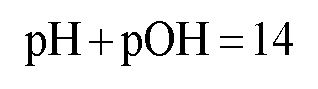Example #1: What is the hydroxide ion and hydronium ion concentration of an aqueous solution that has a pH equal to 6.12?

Transcript

Hey guys, in this new video, we’re going to put to practice some of the concepts we learned about pH and pOH. Let’s take a look at the first example. Here it says what is the hydroxide ion, so what’s the OH-, and the hydronium ion concentration, that’s H+, of an aqueous solution that has a pH equal to 6.12? We have pH. We need to find OH- and H+. Remember we’re able to derive a formula that connects us between H+ and pH. Remember that H+ equals 10 to the negative pH. All we have to do now is take that number and plug it in. When we do that, we get 7.59 times ten to the negative 7.
We could find OH now and we can find OH in two different ways. The first way we could find OH- is by simply using this equation: KW equals H+ times OH-. We know what KW is. We assume that the temperature is 25 degrees Celsius since they don’t tell us what it is. This would be 1.0 times 10 to the negative 14. We just found out what H+ is. It’s 7.59 times 10 to the negative seven. Now we have to find OH-. OH- is our only missing variable so just isolate it. Here, OH- would equal 1.32 times 10 to the negative 8. That would be one way we could find OH-. The second way we could find it is if we know pOH, we can make a connection between pOH and OH-. First, we’d say that pH plus pOH equals 14. We know pH is 6.12, so let’s just isolate our pOH. Subtract 6.12 from both sides so pOH equals 7.88. Remember, what’s the connection between pOH and OH-? You can just simply say that OH- equals 10 to the negative pOH. If you did 10 to the negative 7.88, you get the same exact answer for OH-.
Two different methods to find the same exact missing variable. It’s basically your choice on deciding which way you want to go. Personally, I like just find pOH and just do 10 to the negative pOH because remember, if you don’t put these two in brackets, your calculator may give you the incorrect answer. It’s probably always safer just to say OH- equals 10 to the negative pOH.

Practice: Which of the following solutions will have the lowest concentration of hydronium ions?

Practice: Which of the following statements about aqueous solutions is/are true?

Example #2: A solution is prepared by dissolving 0.235 mol Sr(OH)2 in water to produce a solution with a volume of 750 mL.

a) What is the [OH-]?

b) What is the [H+]?

Practice: What is the Kw of pure water at 20.0°C, if the pH is 7.083?

###### Auto – Ionization Product

Concept #5: Understanding the Auto-Ionization Reaction.

Transcript

Hey guys, in this new video we are going to take a look at the auto ionization of water. So what we’re going to see here is in an aqueous solution so the solvent is water, water molecules can react with each other. And we’re going to say that this is called a process of auto ionization or self-ionization. Now in this process we’re going to create H3O+ and OH-. I just realized, how does this happen? For this to occur, one of the water molecules has to act as an acid and the other one has to act as a base. So let’s say that this one here acts as the acid, and this here is the base. Remember, Bronsted-Lowry definitions, the acid acts as an H+ donor, the base acts as an H+ acceptor.
So this base right here is going to accept an H+ and as a result it’s going to become H3O+. Then we’re going to say that the other water molecule, the one that’s acting as an acid because it’s giving away an H+ it becomes OH-. And just also realize here that water molecules exist in liquid form but then once they become these ions, the hydronium ion and the hydroxide ion, they’re going to be aqueous. They’re no longer liquid water and because of that they’re no longer a liquid they’re going to be aqueous. Remember aqueous just means that the parts of the water are attracted to these particular ions. We can simply auto ionization or self-ionization by just saying that water separates into H+ plus OH-. remember we said this before that H+ is the same thing as H3O+. Both are hydronium ion, they both mean the same exact thing.
Now we’re going to say here that the equilibrium equation for water is called the ion product. An ion product is simply said to be KW. So we aren’t talking about the KW for water, we’re talking about the ion product of water. Now we’re going to say that KW= your hydronium ion multiplied times your hydroxide ion. And we’re going to say here that at 25 degrees Celsius, KW is 1.0 times 10 to the negative 14. But remember just like all K’s it’s going to be temperature dependent. If you’re going to change the temperature you’re going to change the value of the KW. We’re going to say increasing the temperature will increase our KW.
Now here I give us a bunch of different values for KW found at various temperatures. And notice as the temperature gradually increases our KW will get bigger and bigger. Now just realize on your exam when you need to use KW if they don’t give you a temperature, just assume that it’s 25 degrees Celsius. So then KW would be 1.0 times 10 to the negative 14. Knowing this, let’s take a look at this question here.

In a self-ionization reaction two water molecules react with one another, where one acts an acid and the other as a base.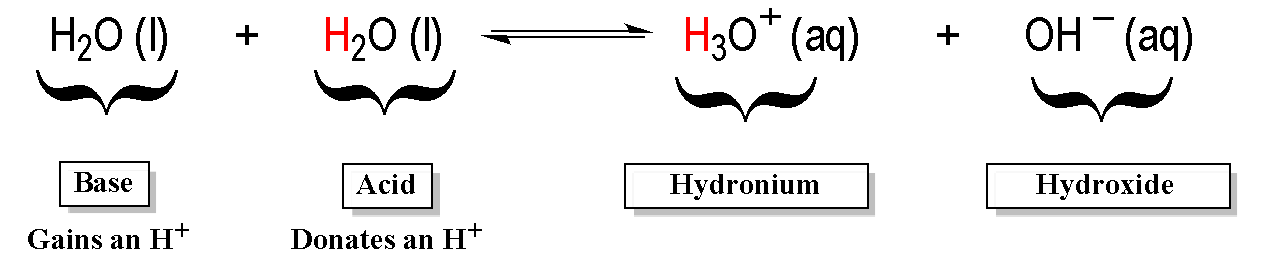The ionization product of Kw is used in this self-ionization equation and ignoring solids and liquids gives the  equilibrium equation as: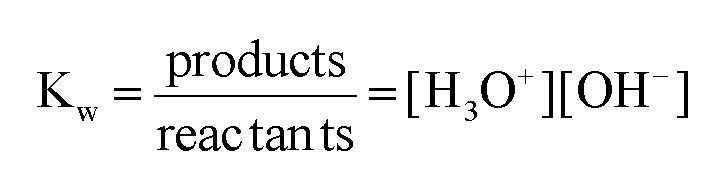Example #3: Determine the concentration of hydronium ions for a neutral solution at 25oC and at 50oC.

Transcript

This question states, determine the concentration of hydronium ion. Basically, find H+ or H3O+. They both mean the same thing. For a neutral solution at 25 degrees Celsius and 50 degrees Celsius.
What we should realize here is what does neutral mean. Neutral means that your compound is not acidic or basic. And what it really means is that H3O+ equals OH-. They’re both the same thing. And because they’re equal to each other, we’re going to set them both to an unknown variable. We’re going to set them to x. Then what we’re going to do here is we know at 25 degrees Celsius KW will be 1.0 times 10 to the negative 14. So at 25 degrees Celsius that’s what our KW is. And we just said that H3O+ and OH- are equal when the solution is neutral and we’re going to set them both equal to x. So then you’re going to say x times x really means x squared. Now you only want to find x, not x squared so take the square root. In this case, x=1.0 times 10 to the negative 7. That number right there represents my concentration of H3O+ and technically because it’s neutral, it also represents the concentration of OH-. If you wanted to do it at 50 degrees Celsius, we’d have to realize that since the temperature changes, KW changes to this new number here. We’re going to say 5.476 times 10 to the negative 14, same exact process, equals x squared. We just want x so we’re going to take the square root of both sides. When we do that, it’s going to give us a final answer of x=2.34 times 10 to the negative seven.
Remember, this is important to know because KW equals H+ and sometimes OH-. This equation is important because if the temperature is not given, assume that KW equals 1.0 times 10 to the negative 14. If you know H+ concentration, then you know OH- concentration because KW will be our constant and vice versa. If you know OH-, you can find H+. This is the relationship we need to understand and these are the beginning steps for us to figure out the pH and pOH of different types of aqueous solutions.

###### pH of Strong Acids and Bases

If we have a STRONG ACID or STRONG BASE then we DO NOT use an ICE chart to find the pH of solution because the yield of H+ and OH  ions are known.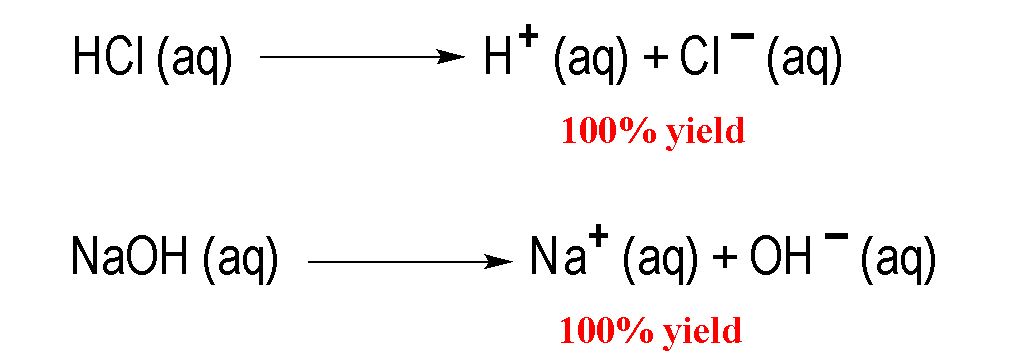Concept #6: Calculating the pH of a Strong Acid or Strong Base.

Transcript

Hey guys, in this new video, we're going to take a look at the connection between pH and pOH and how they relate to strong acids and strong bases. Remember, we've talked about this before. We're going to say that strong acids and strong bases are considered to be strong electrolytes. Remember, strong electrolytes completely ionize. That means they break up a hundred percent. You may even hear that these strong acids and bases are called highly electrolytic. That also means they break up completely, highly electrolytic. If we take a look here, we have HCl and NaOH. Remember, from the rules we've learned, we know HCl is a strong binary acid and we know NaOH is a strong base because we have a group 1A ion, Na+ connected to OH-. What happens here, we have a single arrow going forward because they break up a hundred percent to give us these ions.
We'd say that the product side is highly favored. Just realize, if we have a strong acid or a strong base, I’ve said it before, we don't have to use an ICE chart in order to find pH or pOH. If you have a strong acid, take the negative log to find pH. If you have a strong base, take the negative log to find pOH. Let's take a look at example 1.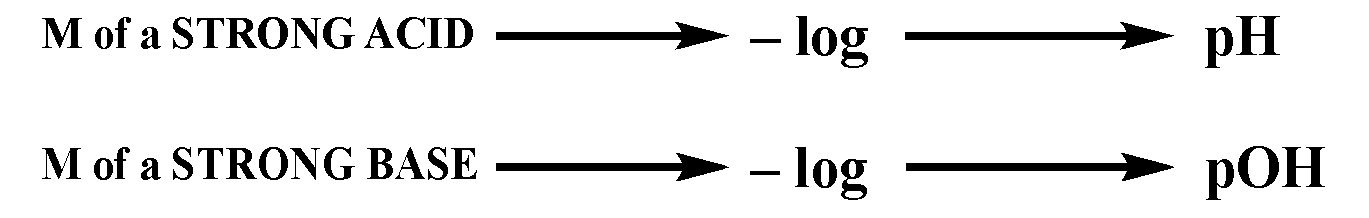Example #4: Calculate the pH of a 0.0782 M solution of CaH2.

Transcript

In example 1, it says: Calculate the pH of a 0.0782 molar solution of calcium hydride. We know that calcium hydride is a strong base, because remember, we have calcium which is Ca2+ connecting with H-, our hydride ion. What happens here? The two from here came here, the one from here came here. That's how we got calcium hydride. What we should realize here is that there are two calcium hydrides in the formula. Now, this is what you need to remember when it comes to strong bases. For strong bases, you have to be very careful. We're going to say that this is not the real concentration of our strong base. You're going to say that when you have strong bases, you have to look at the number of OH-, H-, NH2-, O2-. You have to look at to see how many of each you have in order to determine the real concentration of that strong base. In this particular question, we have two H-. Because we have two of them, we have to multiply this by two. That will give us the correct concentration of our strong base. You have to remember that.
For example, if I had COH2 and there was 0.100 molar of it, again because you have two OHs, you’d have to multiply that by two. If you had calcium amide, you would have two NH2, so again you’d have to multiply the concentration times two to get the correct concentration. Now what we're going to do is we're going to multiply the initial concentration times two to get the real concentration. When we do that, we're going to get our correct concentration. That's the number that we're going to plug in. When we do that, and I'm going to remove myself guys from the image so we have more room to work with. When we do that, we’re going to get a new number, a new value for our OH- concentration.
Here we're going to get 0.1564 molar. That becomes our new concentration. We're going to say pOH equals negative log of OH-. It becomes negative log of 0.1564 molar. Some of you may be asking, “But Jules, this is saying the concentration of OH-. We don't have OH-. We have H-. How do they relate?” Just realized that all four of these ions can be treated as being the same. They all feed into the concentration of OH-. OH- is just understood as a general term for these strong basic ion of a strong base. If I have two OH-, that's equivalent to two H-, equivalent to two NH2-. The same thing. When we take the negative log of this, this will give us our pOH. It’s going to give us 0.814 for our pOH.
But remember, let's pay attention. I didn't ask for the pOH, I asked for the pH. We need to use the next equation, pH plus pOH equals 14. Plug in what we know for pOH, 0.81. We need pH so subtract 0.81 from both sides. So pH here equals 13.19. So that will be our pH for this first question.
Hopefully you guys didn't get lost but what I was saying. Just remember, for strong bases you have to look to see how many OH- you have, H- you have, NH2- you have and O2- you have. Their number determines the correct concentration of our strong base. Right now, what you need to realize is we only do this for strong base at this point. If we had a strong acid such as H2SO4, that strong acid has two acidic Hs. But we wouldn't need to multiply the concentration times two because when it comes to acids, the first H coming off is extremely strong. But the second one that comes off is extremely weak. Because the second H+ is extremely weak, we wouldn't need to multiply the concentration times two. We only do this multiplication of concentrations when we have strong bases. Later on when we deal with acid-base titrations and buffers, we'll talk about how acids play a role.

Example #5: Calculate the pH of a 0.000550 M HBr solution to the correct number of significant figures.

1. 3.3
2. 3.26
3. 3.260
4. 3.2596
5. All are correct

Practice: Calculate the pH of 50.00 mL of 4.3 x 10-7 M H2SO4.

###### pH of Weak Acids and Bases

If we have a WEAK ACID or WEAK BASE then we DO use an ICE chart to find the pH of solution because the yield of H+ and OH  ions are unknown.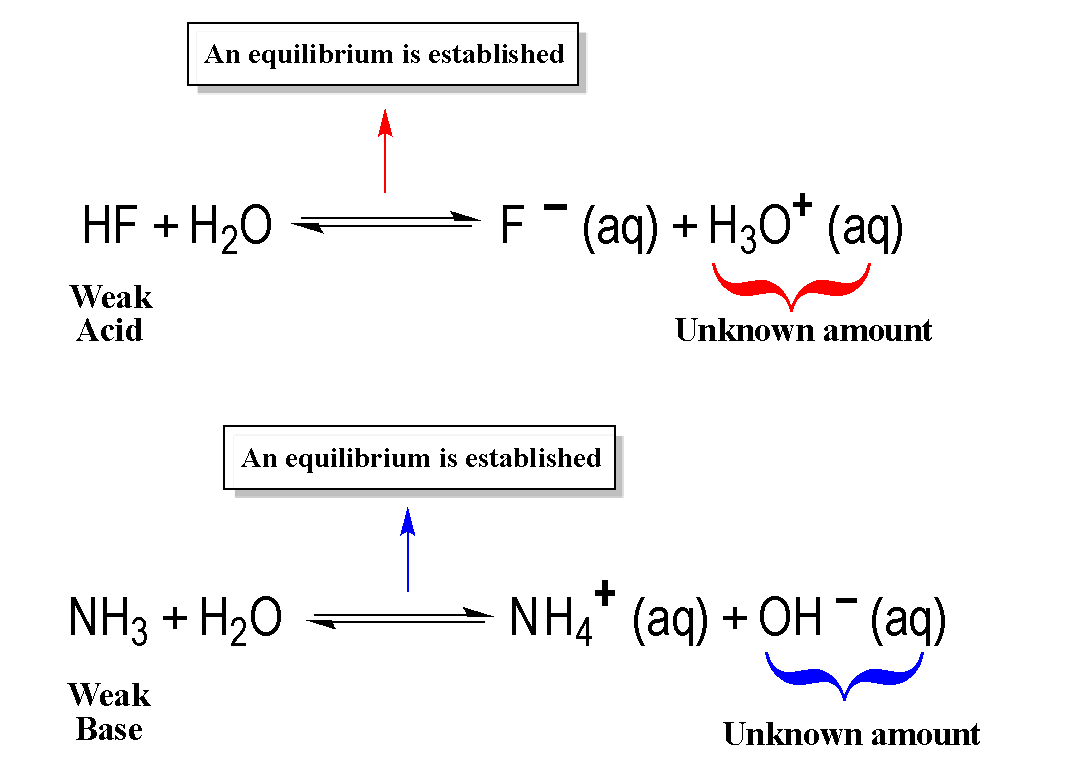Concept #7: Calculating the pH of a Weak Acid or Weak Base.

Transcript

Hey guys, in this new video, we're going to take a look at how exactly do we calculate the pH or pOH of a weak species. Remember we've talked about this before. We're going to say that weak acids and bases are weak electrolytes, which means they don't completely break up when they dissolve in water. What you should notice here that we're going to have double arrows because instead of going to completion, they're going to establish an equilibrium. We're going to say here for weak acids and weak bases, the reactant side is highly favored which means that our compounds stay mostly as reactants. Very little of it breaks down to give us products. We’re going to say the reactant side is highly favored. Majority of our compound will stay as reactants.

To determine the pH of a weak acid or weak base we must use an ICE Chart.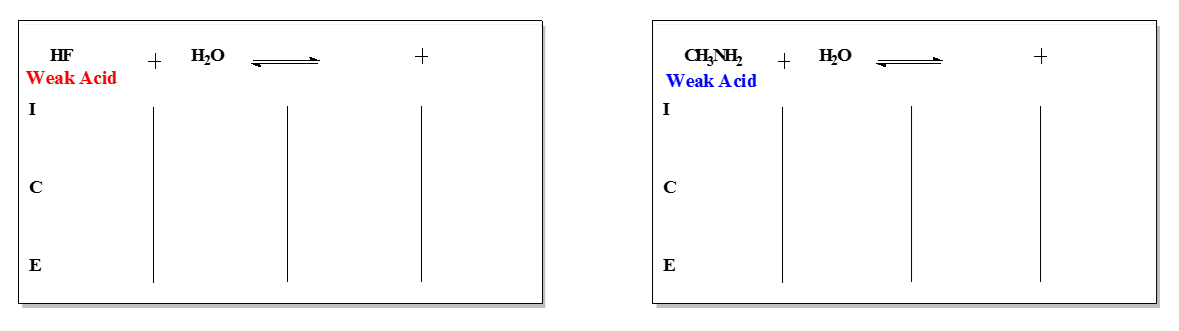Example #6: Pryridine, an organic molecule, is a very common weak base.

C5H5N (aq) + H2O (l)  ⇌  C5H5NH+ (aq) + OH- (g)

Assume you have a 0.0225 M aqueous solution of pyridine, C5H5N, determine its pH. The Kb value for the compound is 1.5 x 10-9

Transcript

Let's take a look at this first question here. It says: Pyridine, an organic molecule, is a very common weak base. We should know that it's a weak base because if we take a look at pyridine, it has carbon, hydrogen and nitrogen. Remember, that's an indication that we have an amine. It doesn't have a positive charge on it so it's a neutral amine which would make it a weak base. Again, the rules are incredibly important to remember. In this question, I make it really easy for us. I tell you it's a weak base. But expect your professors not to be that obvious about it. They'll give you a compound and it's up to you to identify it as either an acid or a base, weak or strong.
Alright, so we have a weak base here. It says: Assume you have a 0.0225 molar solution of pyridine. Determine its pH. The Kb value for the compound is 1.5 times 10 to the negative 9. Now, we're going to need a lot of room to work this first ICE chart out. I'm going to remove myself from the image guys so we have more room to work with.
So we know we have a weak base and I told you, I’ve said it multiple times, if you have a weak acid or a weak base, we have to use an ICE chart. Let's set up this ICE chart. We're going to say C5H5N plus water. I'm just rewriting the equation we have above. We know this has to be the equation because remember, if this is the base then the water has to be the acid. The water has to give away an H+ to the base. The base accepts the H+ and that's how it became this conjugate acid. The water loses an H+ and that's how it becomes this conjugate base. Now, we're going to say we're going to do an ICE chart. So it’s initial, change, equilibrium. That's what ICE stands for. Remember, in an ICE chart we ignore two phases. We ignore solids and liquids. The water is a liquid so we're going to ignore it. Again, we ignore solids and liquids. Next we're going to say since this is an ICE chart dealing with acids and bases, we have to have the units in molarity. The good thing is I already gave you the units and it is molarity. If they didn't give it to you in molarity, you’d have to make sure you convert them to that.
I don't tell you anything initially about the products so assume they’re zero. Now, here's the important thing. The important sense you need to remember for acid and base reactions. We lose reactants to make products. We're losing the reactant so it's going to be negative x because we don't know what the change is. Remember, it's weak. We don't really know how much of it is breaking down. If we don't know how much of it is breaking down, we don't know how much product we’re making. This is going to be plus x because their products were making them. Bring down everything. So, this is going to be 0.0225 minus x plus x plus x. We’ve just filled out our first ICE chart for a weak base.
Now what we're going to say is since it's a weak base, bases use Kb. Here we're going to say Kb equals products over reactants. I told you the Kb value right here. It's 1.5 times 10 to the negative 9. Let's just plug in what we know. This would be 1.5 times 10 to the negative 9. Then we're going to have the products multiply. Just write the equilibrium expression here. So, this is the equilibrium expression we have. These two things are x in here and in here. x times x is x squared divided by 0.0225 minus x.
Now, here's the crappy thing about ICE charts. Sometimes we have to use the quadratic. The good thing is when it's acid and base chemistry, we can use a shortcut to see if we can ignore this minus x. If we can ignore that minus x, we don't have to do the quadratic.
To figure out if we could ignore it or not, we're just simply going to do this. We're going to take our initial concentration. Our initial concentration is this number and divide it by our k value, whichever k we’re using. In this case we happen to be using Kb. It’d be over Kb. But if we use another question and the value had to be Ka, then it will be the initial divided by Ka. We're going to say if you’d get an answer
greater than 500, then you can ignore the minus x. Just remember that this is the trick we're going to use to see if we can ignore that minus x. Take the initial concentration, divide it by the K value were using. If you get an answer back greater than 500, that means we could ignore that minus x because that minus x is going to be so small it doesn't affect our real answer.
We're going to take the initial concentration which is 0.0225 and divide it by the K value, 1.5 times 10 to the negative 9. When we do that, we get 1.5 times 10 to the 7. That answer is incredibly large, much bigger than 500, which would mean we could ignore this minus x. This is going to make our Math a lot easier because now we don't have to do the quadratic. What we're going to do here is we’re going to multiply both sides by 0.0225. We're going to say now x squared equals 3.375 times 10 to the negative 11. We don't want x squared, we just want x so take the square root. x here equals 5.81 times 10 to the negative 6.
When it comes to acid-base chemistry, when you find x, x gives you either OH- or H3O+. It's all based on which one is in your equation. In our equation, we have H+. At equilibrium, H plus equals x. What we just found here is the concentration of OH-. If we know the concentration of OH-, we know the pOH because remember, pOH equals negative log of OH-. Plug it in. Here we're going to say here that means that our pOH equals 52.4 but I didn’t ask for pOH. I asked for pH, so now you're going to say pH plus pOH equals 14. pH equals 14 minus pOH. It equals 14 minus 5.24. Your answer here would be 8.76. I know it looks like a lot of work and it is. You have to be very careful with all the Math that you're doing. One little mistake could greatly affect your answer. But this is the way we would set up our ICE chart.
There's going to be a few variations of different types of questions they can ask. But don't worry, the other videos will cover different varieties of ICE charts. This is just our first one. Hopefully you guys didn't get lost along the way as we did this. If you got a little confused here and there, feel free if you have to, post a question dealing with it, if you have any questions on this one or just go back and look at the video again. We know we had a weak base so we had to do this. The thing is, weak bases produce OH- as a product, so we have to change our OH- into pOH, then from pOH to pH. If we started out with the weak acid, it will produce H3O+. We find x and that will give us H3O+ and then you simply, to find pH, take the negative log of H3O+ and that will give us pH. Hopefully guys, you guys get really good at these because it does take practice. Make sure you pay attention to all the steps that we're doing.

Example #7: An unknown weak base has an initial concentration of 0.750 M with a pH of 8.03. Calculate its equilibrium base constant.

Practice: Determine the pH of a solution made by dissolving 6.1 g of sodium cyanide, NaCN, in enough water to make a 500.0 mL of solution. (MW of NaCN = 49.01 g/mol). The Ka value of HCN is 4.9 x 10-10.